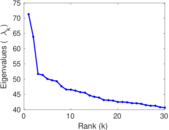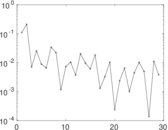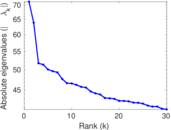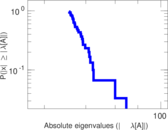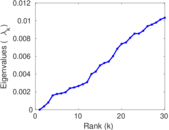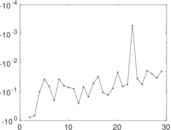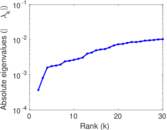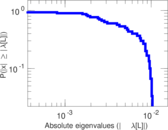# Teams

The is the bipartite network of athletes and their teams, extracted from DBpedia. The nodes in the network are individual athletes and individual teams. An edge in the network connects an athlete with a team the athlete has played in. The dataset was extracted from DBpedia and corresponds to the <http://dbpedia.org/ontology/team> relationship type.

 Code `TM` Internal name `dbpedia-team` Name Teams Data source http://wiki.dbpedia.org/Downloads AvailabilityDataset is available for download Consistency checkDataset passed all tests Category Affiliation network Dataset timestamp 2001 ⋯ 2017 Node meaning Athlete, team Edge meaning Membership Network formatBipartite, undirected Edge typeUnweighted, no multiple edges

## Statistics

 Size n = 935,627 Left size n1 = 901,166 Right size n2 = 34,461 Volume m = 1,366,466 Wedge count s = 336,279,376 Claw count z = 117,855,347,259 Cross count x = 42,549,380,095,569 Square count q = 10,778,376 4-Tour count T4 = 1,434,091,924 Maximum degree dmax = 2,671 Maximum left degree d1max = 17 Maximum right degree d2max = 2,671 Average degree d = 2.920 96 Average left degree d1 = 1.516 33 Average right degree d2 = 39.652 5 Fill p = 4.400 14 × 10−5 Size of LCC N = 876,025 Diameter δ = 41 50-Percentile effective diameter δ0.5 = 5.632 65 90-Percentile effective diameter δ0.9 = 7.691 47 Median distance δM = 6 Mean distance δm = 6.370 73 Gini coefficient G = 0.645 816 Balanced inequality ratio P = 0.247 298 Left balanced inequality ratio P1 = 0.395 676 Right balanced inequality ratio P2 = 0.149 957 Relative edge distribution entropy Her = 0.853 592 Tail power law exponent γt = 1.771 00 Tail power law exponent with p γ3 = 1.771 00 p-value p = 0.000 00 Left tail power law exponent with p γ3,1 = 8.991 00 Left p-value p1 = 0.000 00 Right tail power law exponent with p γ3,2 = 1.771 00 Right p-value p2 = 0.000 00 Degree assortativity ρ = +0.050 656 0 Degree assortativity p-value pρ = 0.000 00 Spectral norm α = 71.312 4 Algebraic connectivity a = 0.000 368 766 Spectral separation |λ1[A] / λ2[A]| = 1.115 96 Controllability C = 868,811 Relative controllability Cr = 0.928 623

## Plots

### Fruchterman–Reingold graph drawing### Degree distribution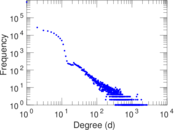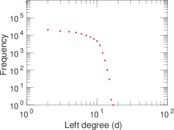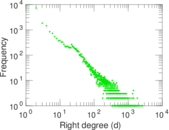### Cumulative degree distribution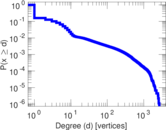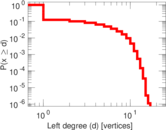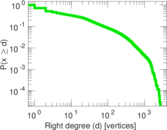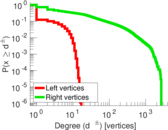### Lorenz curve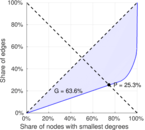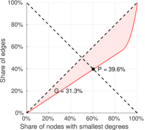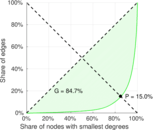### Spectral distribution of the adjacency matrix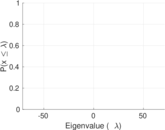### Spectral distribution of the normalized adjacency matrix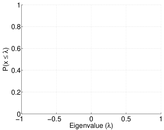### Spectral distribution of the Laplacian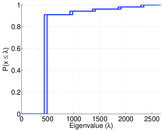### Spectral graph drawing based on the adjacency matrix### Spectral graph drawing based on the Laplacian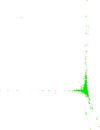### Spectral graph drawing based on the normalized adjacency matrix### Degree assortativity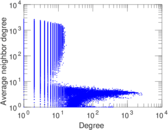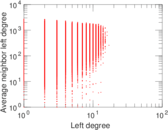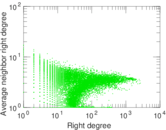### Zipf plot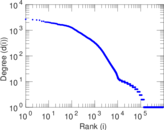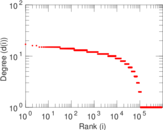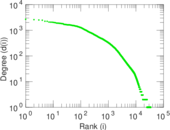### Hop distribution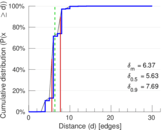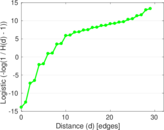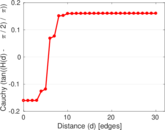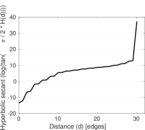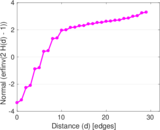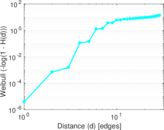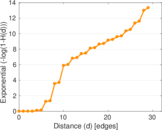### Delaunay graph drawing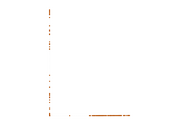### Matrix decompositions plots